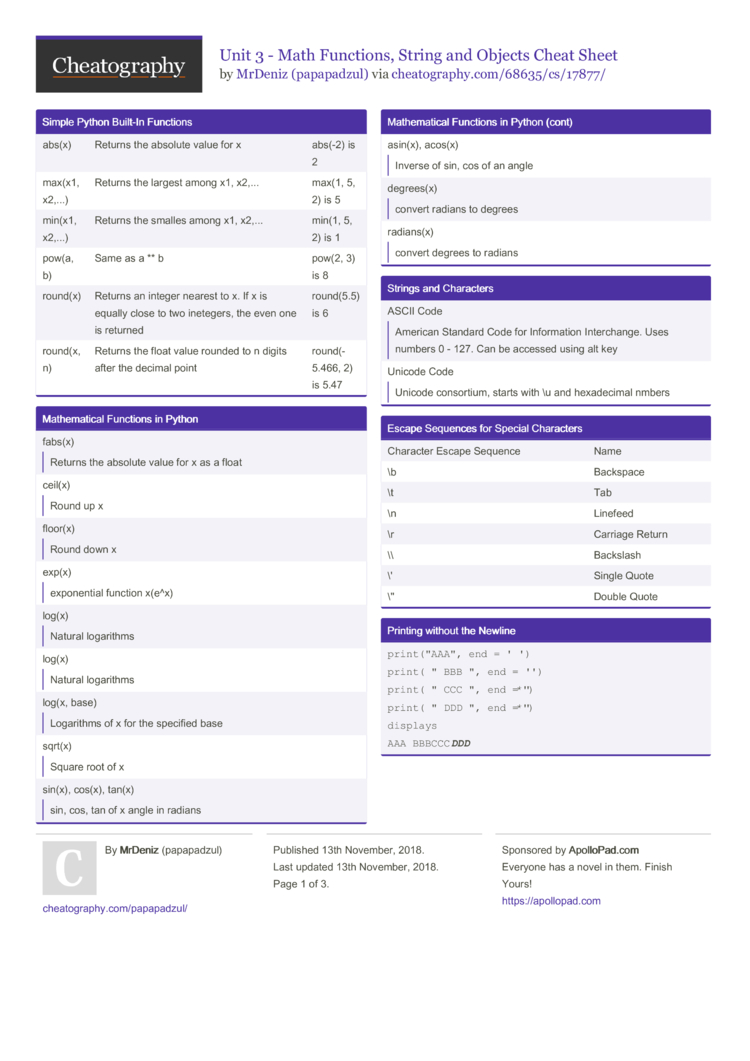# Unit 3 - Math Functions, String and Objects Cheat Sheet by papapadzul

### Simple Python Built-In Functions

 abs(x) Returns the absolute value for x abs(-2) is 2 max(x1, x2,...) Returns the largest among x1, x2,... max(1, 5, 2) is 5 min(x1, x2,...) Returns the smalles among x1, x2,... min(1, 5, 2) is 1 pow(a, b) Same as a ** b pow(2, 3) is 8 round(x) Returns an integer nearest to x. If x is equally close to two inetegers, the even one is returned round(5.5) is 6 round(x, n) Returns the float value rounded to n digits after the decimal point round(­5.466, 2) is 5.47

### Mathem­atical Functions in Python

 fabs(x)Returns the absolute value for x as a float ceil(x)Round up x floor(x)Round down x exp(x)expone­ntial function x(e^x) log(x)Natural logarithms log(x)Natural logarithms log(x, base)Logarithms of x for the specified base sqrt(x)Square root of x sin(x), cos(x), tan(x)sin, cos, tan of x angle in radians asin(x), acos(x)Inverse of sin, cos of an angle degrees(x)convert radians to degrees radians(x)convert degrees to radians

### Strings and Characters

 ASCII CodeAmerican Standard Code for Inform­ation Interc­hange. Uses numbers 0 - 127. Can be accessed using alt key Unicode CodeUnicode consor­tium, starts with \u and hexade­cimal nmbers

### Escape Sequences for Special Characters

 Character Escape Sequence Name \b Backspace \t Tab \n Linefeed \r Carriage Return \\ Backslash \' Single Quote \" Double Quote

### Printing without the Newline

 ```print("AAA", end = ' ') print("BBB", end = '') print("CCC", end = '*') print("DDD", end = '*') displays AAA BBBCCCDDD```

### Invoke the print function with the end argument

 ```n = 3 id(n) type(n) s = salalah sl = s.lower() print (s) --- su = s.upper() print(s) s = “\t Salalah \n” print(s) s = s.strip() print(s)```

### Convert numbers to a string

 ```num1 = str(3.4) print (num1) num2 = str(5) print (num2)```

### Read strings from the keyboard

 ```firstname = input(“firstname is: “) secondname =input(“second name is: “) name = firstname + secondname print (name) print(firstname + “ “ + secondname)```

### To use the + operator to concat­enate strings

 ```firstname = “ Mohammad” secondname = “Ali” name = firstname + secondname print (name) Firstname + = secondname print(firstname) use “ “ space```

### Format Strings and Numbers

 ```a = 3 b = .123 c = a / b print(c) --- print (round(c,2)) --- print (format(c,”10.2f”)) print (format(c,”10.2e”)) print (format(c,”10.%”) --- (for int only) print(format(a,”b”)) #binary print(format(a,”o”)) #octal print(format(a,”x”)) #hexadecimal```

### Format Strings and Numbers cont...

 ```print (format(c,”10.2f”)) print (format(c,”<10.2f”)) print (format(c,”>10.2f”) --- print(format(a,”b”)) print(format(a,”b”)) --- s = "I love Python" print(s) print(format(s,"20s")) print(format(s,"<20s")) print(format(s,">20s"))```

### Draw Various Shapes

 ```import turtle turtle.pensize(n) 1-3 turtle.penup() turtle.goto(x,y) (-200,-50) turtle.pendown() turtle.circle(40, steps = 3) #triangle turtle.done()```

### Draw graphics with colors and fonts

 ```import turtle turtle.pensize(n) turtle.penup() turtle.goto(x,y) turtle.pendown() turtle.begin_fill() turtle.color(“color”) turtle.circle(40, steps = 3) turtle.end_fill() turtle.done()```

## Download the Unit 3 - Math Functions, String and Objects Cheat Sheet2 Pages

PDF (recommended)